# Cauchy Schwarz inequality

(diff) ← Older revision | Latest revision (diff) | Newer revision → (diff)

The Cauchy inequality for finite sums of real numbers is the inequality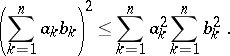Proved by A.L. Cauchy (1821); the analogue for integrals is known as the Bunyakovskii inequality.

The Cauchy inequality is also the name used for an inequality for the modulus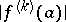of a derivative of a regular analytic function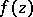at a fixed point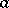of the complex plane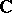, or for the modulus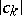of the coefficients of the power series expansion of,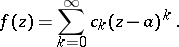These inequalities are(*)

whereis the radius of any disc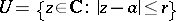on whichis regular, and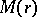is the maximum modulus of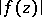on the circle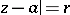. The inequalities (*) occur in the work of A.L. Cauchy (see e.g. ). They directly imply the Cauchy–Hadamard inequality (see ):where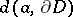is the distance fromto the boundaryof the domain of holomorphy of. In particular, ifis an entire function, then at any point,For a holomorphic functionof several complex variables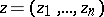,, the Cauchy inequalities are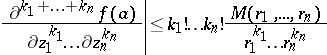orwhere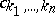are the coefficients of the power series expansion of: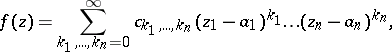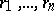are the radii of a polydisc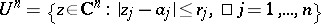on whichis holomorphic, and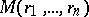is the maximum ofon the distinguished boundary of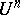.

The distinguished boundary of a polydiscas above is the set.